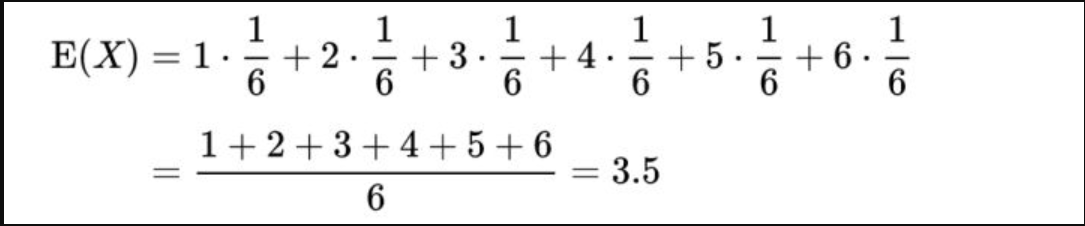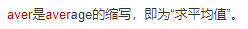# -1.灌水

• 想了解更多关于数论的内容，可戳这里# 2、期望的小性质：

• 设X是随机变量，C是常数，则$E(CX)=C\times E(X)$

$$Ca_1,Ca_2,Ca_3...Ca_n$$

$$p_1,p_2,p_3...p_n$$

$$E(CX)=C\sum_{i=1}^{n}(a_i\times p_i)$$

(C提出来)

$$E(X)=\sum_{i=1}^{n}(a_i\times p_i)$$

$$E(CX)=C\times E(X)$$

• 设X，Y是任意两个随机变量，则有$E(X+Y)=E(X)+E(Y)$。

• 设X，Y是相互独立的随机变量，则有$E(XY)=E(X)\times E(Y)$。

• 设C为常数，则$E(C)=C$。

# 3.期望与均值？

• 均值往往是在实验中简单的对数据进行平均。

• 而期望就好像在上帝视角的人。

$$\frac{1+5+5+6+3+3}{6}=3.8333333...$$# 4.引入：

50%:甲赢，拿下100元。

50%：乙赢，继续比赛。

### 但是，如果问题就进行到这里，也就没有接下来的期望了。

$\frac{1}{2}\times \frac{1}{2}=\frac{1}{4}$

### 问题2：

#### 所以就要换喽# 5、期望的应用

### 彩票问题

• 末位相等,安慰奖：奖励4元，中奖概率0.1

• 后两位相等,幸运奖：奖励20元，中奖概率0.01

• 后三位相等,手气奖：奖励200元，中奖概率0.001

• 后四位相等,一等奖：奖励2000元，中奖概率0.0001

• 后五位相等,特等奖：奖励20000元，中奖概率0.00001

$$0.1\times 4+0.01\times 20+0.001\times 200+0.0001\times 2000+0.00001\times 20000=1.2$$dalao:# 6.例题1

https://www.luogu.org/problem/P2911

• 题意简叙：

### 解法2：

$$\frac{(a+b+c+3)}{2}$$

# 7.例题2：

tag：这道题笔者并没有找到题目出处，如有发现者，欢迎在评论区留言！

• 给定一个无向图，每个点可以等概率地走到与它有边的点

• 求从1走到n所需要的期望步数

• N<=500

### 分析：

• $F[i]$表示从i走到n的期望步数

• $F[n]=0$;

• $F[i]=aver${$f[j]$}$+1$,$(i,j)$有边

tag：• 构成n元一次方程组

• 高斯消元？

# 8.例题3

https://www.luogu.org/problem/UVA10288

• 题意简叙：

$n\leq33$

### 分析：

$$a=\frac{k}{n}$$

$$a^{t-1}(1-a)$$

$$(1-a)(1+2a+3a^2+4a^3+5a^4+...)$$

$$E(1-a)$$

$$E(a)=a+2a^2+3a^3+4a^4+...=E-1-a-a^2-a^3...$$

$$E(1-a)=1+a+a^2+a^3=\frac{1}{1-a}$$

$$E(1-a)=\frac{n}{n-k}$$

$$(\frac{1}{n}+\frac{1}{n-1}+\frac{1}{n-2}+\frac{1}{n-3}+\frac{1}{2}+1)n$$

# 9.例题4：

• 题意简叙：

## 分析：

$$a[i]=(a[i-1]+1)\times p[i]$$

$tag$：即为在i-1的末尾加一个概率为$p[i]$出现的1

$$b[i]=(b[i-1]+2\times a[i-1]+1)\times p[i]$$

$tag$:期望的线性延伸：

$$x^2->(x+1)^2->x^2+2x+1$$

$$f[i]=(f[i-1]+3\times b[i-1]+3\times a[i-1]+1)\times p[i]$$

• 哇塞我要A紫题了！！！$$f[i]=(f[i-1]+3\times b[i-1]+3\times a[i-1]+1)\times p[i]+f[i-1]\times (1-p[i])=f[i-1]+(3\times b[i-1]+3\times a[i-1]+1)\times p[i]$$

### code：

//AC记录：https://www.luogu.org/record/21569138
#include<cstdio>
using namespace std;
double a,b,f,p;
int main()
{
int n;
scanf("%d",&n);
for(int i=1;i<=n;i++)
{
scanf("%lf",&p[i]);
a[i]=(a[i-1]+1)*p[i];
b[i]=(b[i-1]+2*a[i-1]+1)*p[i];
f[i]=f[i-1]+(3*b[i-1]+3*a[i-1]+1)*p[i];
}
printf("%.1lf\n",f[n]);
return 0;
}$tag:$这题还可以扩展到k次，也就是$X^k$，用二项式定理$O(nk^2)$解决

# 10、例题5

https://www.luogu.org/problem/P4550

• 题意简叙：

n个数1~n，第k次取数需要k元，每次取数对于所有数概率均等（$\frac{1}{n}$）,问取完n个数的期望花费

### 分析：

$$\sum_{i=1}^{x}i=\frac{(x^2+x)}{2}$$

$a[i]$表示找完i个数之后还需要的次数的期望

$f[i]$表示找完i个数之后还需要的次数平方的期望

### 先来考虑$a[i]$

$$a[i]=?$$

#### 情况2，买到没买过的

$$a[i]=\frac{i}{n}(a[i]+1)+\frac{n-i}{n}(a[i+1]+1)$$

……&%&（）……&……&%……&%……*&%

$$a[i]=a[i+1]+\frac{n}{n-i}$$

### 然后考虑$f[i]$

$$f[i]=\frac{i}{n}(f[i]+2\times a[i]+1)+\frac{n-i}{n}(f[i+1]+2\times a[i+1]+1)$$

• 倒推
• 边界$f[n]=0$
• 可算，但麻烦
• 化简

OK既然上面讲了写式子下面就说说化简的事吧~

$$f[i]=\frac{i}{n}(f[i]+2\times a[i]+1)+\frac{n-i}{n}(f[i+1]+2\times a[i+1]+1)$$ 把第一个括号拆成$f[i]$和$2\times a[i]+1$两部分

$$\frac{n-i}{n}f[i]=\frac{i}{n}(2\times a[i]+1)+\frac{n-i}{n}(f[i+1]+2\times a[i+1]+1)$$

$$f[i]=\frac{i}{n-i}(2\times a[i]+1)+f[i+1]+2\times a[i+1]+1$$

end

### code：

#include<cstdio>
using namespace std;
double a,f;
int main()
{
int n;
scanf("%d",&n);
a[n]=0;
f[n]=0;
for(int i=n-1;i>=0;i--)
{
a[i]=a[i+1]+1.0*n/(n-i);
f[i]=1.0*i/(n-i)*(2*a[i]+1)+f[i+1]+2*a[i+1]+1;
}
printf("%.2lf\n",(f+a)/2);
return 0;
} 

# 11、其他推荐题目

P1850 换教室 ————2016NOIPTG题目，dp+期望，值得一做 P3802 小魔女帕琪————也挺好，入门的分析